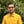Trusted answers to developer questions
Trusted Answers to Developer Questions

Related Tags

swift
exp
math
communitycreator

# What is the exp() function in Swift?Harris Amjad

Grokking Modern System Design Interview for Engineers & Managers

Ace your System Design Interview and take your career to the next level. Learn to handle the design of applications like Netflix, Quora, Facebook, Uber, and many more in a 45-min interview. Learn the RESHADED framework for architecting web-scale applications by determining requirements, constraints, and assumptions before diving into a step-by-step design process.

### Overview

In Swift, the exp() function returns the mathematical e raised to the power of a specific number. Here, Euler’s number e is the base of the natural logarithm.

The mathematical representation of the exp() function is shown below:

Mathematical representation of the exp() function

Note: We need to import Foundation in our code to use the exp() function. We can import it like this: import Foundation.

### Syntax

// number should be float, double or real.exp(number)
Syntax of exp() function

### Parameters

This function requires a number as a parameter.

### Return value

This function returns the mathematical e raised to the power of number sent as a parameter.

### Example

The code below shows the exp() function in Swift:

import Swiftimport Foundation// Positive Integerprint ("The value of exp(4.0) : ", exp(4.0));//zeroprint ("The value of exp(0.0) : ", exp(0.0));// Negative Integerprint ("The value of exp(-25.0) : ", exp(-25.0));//Positive double valueprint ("The value of exp(4.4) : ", exp(4.4));//Negative double valueprint ("The value of exp(-4.5) : ", exp(-4.5));
Example of the exp() function

### Code explanation

• Line 2: We add the Foundation header required for the exp() function.
• Line 4: We calculate the exponent value of the positive integer using exp().
• Line 7: We calculate the exponent value of the zero using exp().
• Line 10: We calculate the exponent value of the negative integer using exp().
• Line 13: We calculate the exponent value of the positive double value using exp().
• Line 16: We calculate the exponent value of the negative double value using exp().

RELATED TAGS

swift
exp
math
communitycreator

CONTRIBUTORHarris Amjad

Grokking Modern System Design Interview for Engineers & Managers

Ace your System Design Interview and take your career to the next level. Learn to handle the design of applications like Netflix, Quora, Facebook, Uber, and many more in a 45-min interview. Learn the RESHADED framework for architecting web-scale applications by determining requirements, constraints, and assumptions before diving into a step-by-step design process.

Keep Exploring

Learn in-demand tech skills in half the time